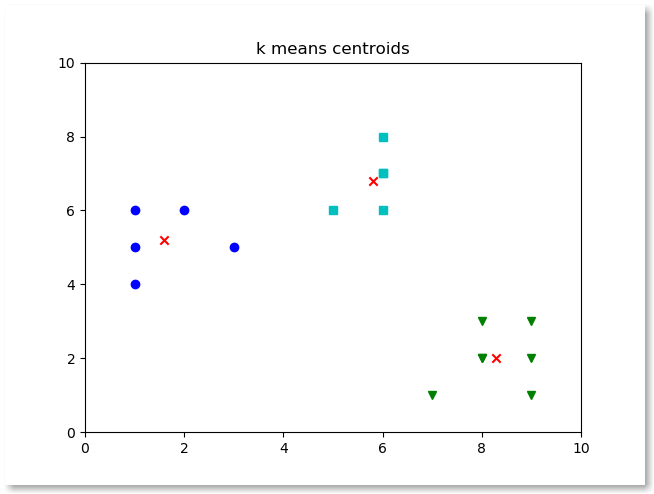The KMeans clustering algorithm can be used to cluster observed data automatically. All of its centroids are stored in the attribute cluster_centers.

Related course: Complete Machine Learning Course with Python

## KMeans cluster centroids

We want to plot the cluster centroids like this:First thing we’ll do is to convert the attribute to a numpy array:

This array is one dimensional, thus we plot it using:

We can plot the cluster centroids using the code below.

If you are new to Machine Learning, I highly recommend this book